# Electronics and Communication Engineering - Digital Electronics

21.

TTL logic is preferred to DRL logic because

 A. greater fan-out is possible B. greater logic levels are possible C. greater fan-in is possible D. less power consumption is achieve

Answer: Option A

Explanation:

No answer description available for this question. Let us discuss.

22.

Assertion (A): Schottky transistors are preferred over normal transistors in digital circuits

Reason (R): A Schottky transistor when used as a switch, between cutoff and active region.

 A. Both A and R are correct and R is correct explanation of A B. Both A and R are correct but R is not correct explanation of A C. A is true, R is false D. A is false, R is true

Answer: Option A

Explanation:

Schottky transistors are fast switching because saturation is avoided.

23.

The inputs A and B of the given figure are applied to a two input NOR gate. The output waveform is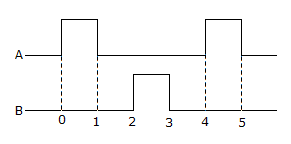A.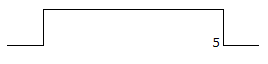B.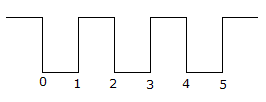C.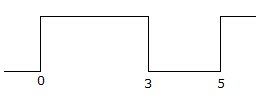D.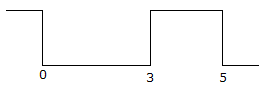Answer: Option B

Explanation:

No answer description available for this question. Let us discuss.

24.

In the circuit of the given figure, V0 =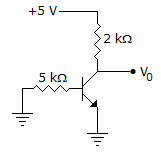A. 5 V B. 3.1 V C. 2.5 V D. 0

Answer: Option A

Explanation:

Transistor is off and circuit current is zero.

25.

A parallel in-parallel out shift register can be used to introduce delay in digital circuits.

 A. True B. False

Answer: Option B

Explanation:

No time delay in this shift register since all bits are loaded and read simultaneously.

#### Current Affairs 2021

Interview Questions and Answers